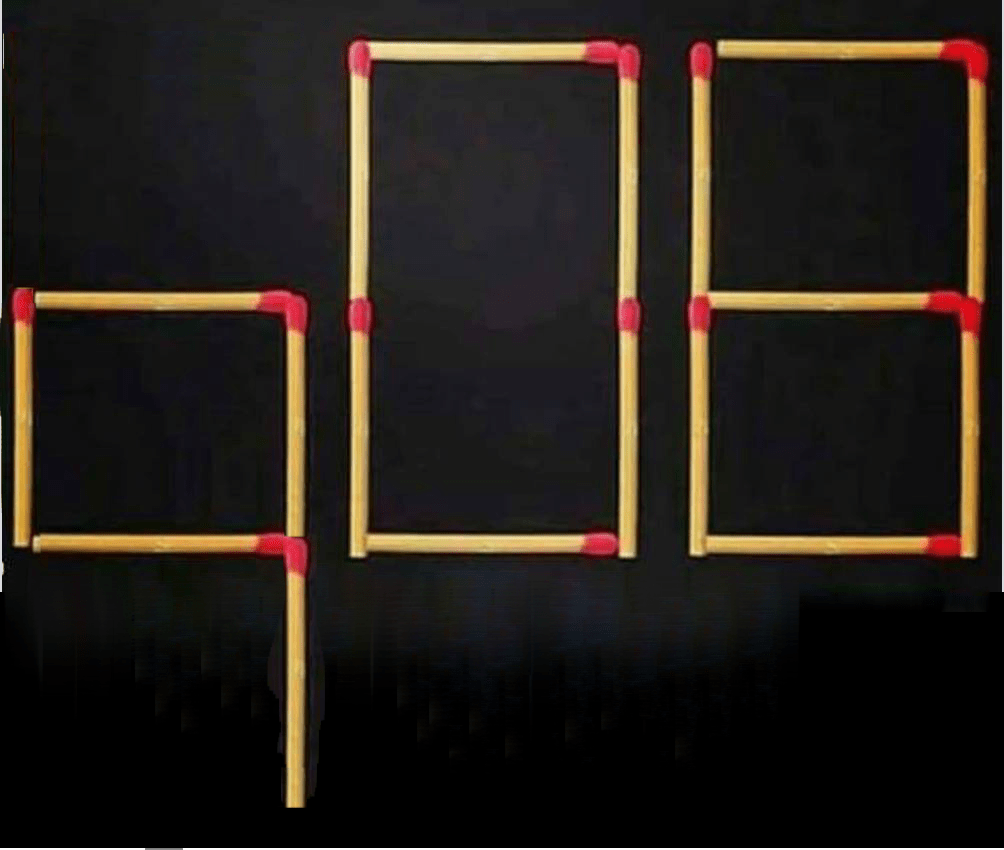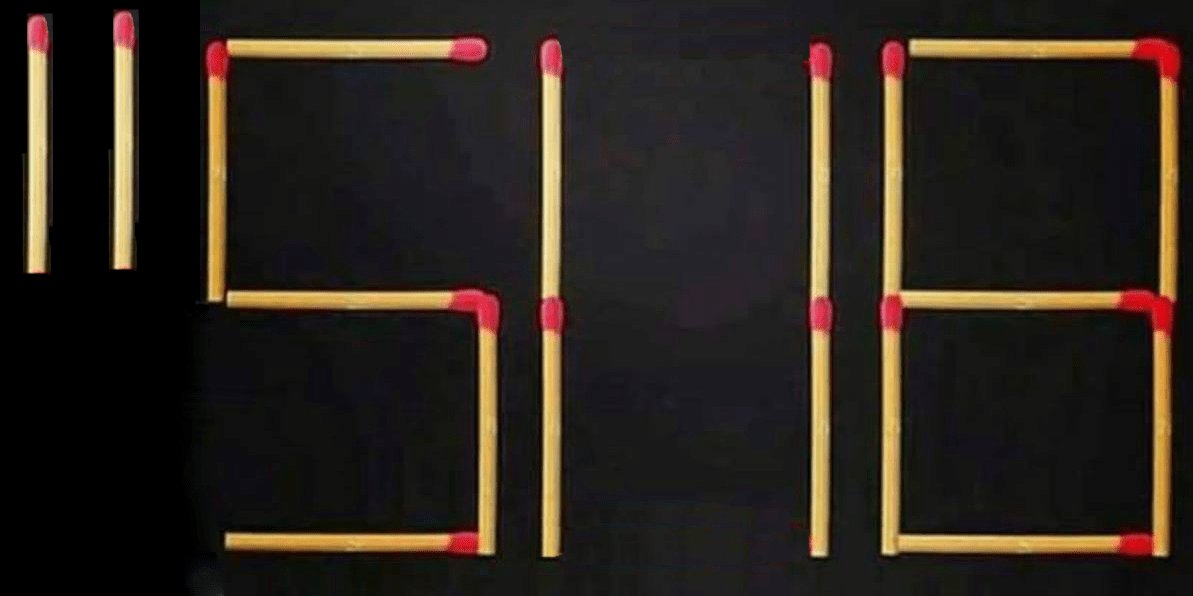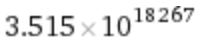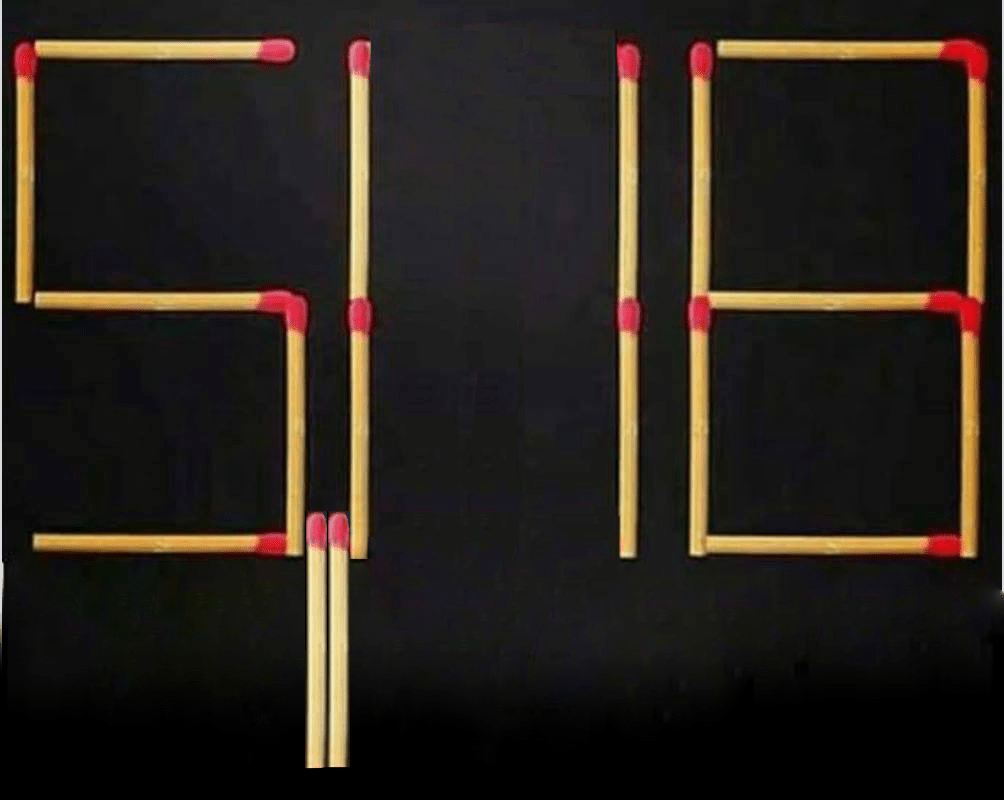# The Match ProblemThis puzzle has been popping up on my Facebook feed recently. Try to see how high you can get before reading on!

(…)

Now, would you be surprised if I told you that with a little interpretive freedom and creativity, you can get numbers larger than the number of atoms in the observable universe? How about if I told you that you can get to numbers large enough that they break set theory? Let me demonstrate for you.

You can move the bottom match in the 5 up to make it a 9. Then you can rotate the bottom left vertical match in the 0 to make it a nine. This gives 998.Can we do better? Sure! Take the top and bottom matches in the central zero and move them to squeeze in another one, giving you 51,118.So far we’ve been working purely in base 10. Let’s try to do better by moving to a more exotic number system.

Hexadecimal is a base-16 number system, the digits of which are written as 0, 1, 2, 3, 4, 5, 6, 7, 8, 9, A, B, C, D, E, and F. For instance, we can construct F88:F88 is only 3976, which is not better than our best so far (51,118). If we’re allowed to interpret a square of matches as a D instead of a zero, we can do slightly better (though to do so we have to be allowed to throw out a toothpick, or place it directly on top of another one):FDB is 4059, which is still not better than our current best. To get better, we need to get more clever.

# Exponentiation!

Our first strategy is just to shift two matches in the first digit down so as to make it into a 9:We can interpret this as 98 = 43,046,721. This is our best so far! But we can do better by applying Knuth’s up-arrow notation.This is 518, which is almost 3.8 trillion, 100 thousand times better than 98! But we’re not done yet! If we use a caret (^) to represent exponentiation, we can get even higher!5118 is 5.4 nonillion, a number with 31 decimal digits long. We could try to do better than this by squeezing in a caret between the 5 and the 1, making 5118 (a number with 83 decimal digits) but this becomes pretty messy and gross.

Alright, so we got up to 83 digit long numbers. Can we get any better? Yep!

# Tetration

Tetration is the level above exponentiation. The nth tetration of a is defined as follows:Just as multiplication is repeated addition and exponentiation is repeated multiplication, tetration is repeated exponentiation! Now we get into numbers whose size is truly impossible to grasp. Let’s shift the top and bottom matches on the middle 0:We can write this equivalently as:How big is this number? There’s really no meaningful way to describe it. We’ve gone far beyond any quantities that can be made physical sense of, like the number of cubic nanometers in the universe, which is a measly 10107. But we’re not done yet!

# Busy Beavers!

The busy beaver numbers are a sequence of numbers that arise from the properties of Turing machines. Here’s a brief description: The nth busy beaver number B(N) is the greatest number of ones that a finitely-running N state Turing machine can print on a tape which starts all zero.

Did you think tetration is big? Well, busy beaver numbers are unimaginably larger. In fact, the busy beaver sequence grows larger than any computable function! There’s a neat proof of this that involves the uncomputability of the halting problem. To skim over it, it can be shown that were we to have an upper bound on the busy beaver sequence, then we could find a deterministic algorithm for solving the halting problem in a finite amount of time, which we know is impossible. And if any computable function F(N) grew faster than B(N), then we could find an upper bound on the busy beaver sequence. Thus, it must be the case that no computable function grows as fast as B(N)!

We can exploit the absurd growth rate of the busy beaver sequence if we are allowed the interpretative freedom of assuming parentheses, so that Bn = B(n).Let’s think for a minute about how large B(118) must be. So far, the only values of n for which B(n) is known are B(1), B(2), B(3), and B(4). After this the values grow out of control. A lower bound on B(6) is. For B(7), we have as a lower bound. B(8) almost certainly beats our current record. And B(118) is unthinkably large.

We can get even higher than the busy beaver numbers with the maximum shifts function S(N), defined as the number of steps that the longest-finitely-running N state Turing machine takes before halting. This function is guaranteed to be larger than B(N) for all N. Using S(N), and taking the same liberties as above with respect to parentheses, we can get an insanely high value:This is S(1118), and while it’s undoubtedly larger than B(118), there’s no way to get any intuitive grasp on how much larger. But wait, there’s more! We can get even larger by recursively nesting a busy beaver function within a maximum shifts function:We interpret this as S(B(9)). Why is this larger than S(1118)? Well, B(9) is some enormous number, certainly larger than 1118, so S(B(9)) is certainly greater than S(1118).

Now, are we finally done? Have we reached the peak yet? No! It’s time for the largest solution of them all.

The reason that the Busy Beaver numbers and Maximum Shift function are so big is because of the uncomputability of the halting problem. But if we consider Turing machines that have an oracle for the halting problem (call these meta Turing machines), we get a new meta-halting problem: when do these meta Turing machines halt? From the meta-halting problem comes an associated new sequence of Busy Beaver numbers, which grows uncomputable faster than the original Busy Beaver sequence. Then we can equip Turing machines with an oracle for the meta-halting problem, generating a meta-meta-Busy Beaver sequence.

Thus we get a hierarchy of Busy Beaver functions, which, following the notation used by Scott Aaronson here, can be described with Bn(x). Each Bn grows uncomputably faster than the previous Bn-1. There’s a similar hierarchy for the maximum shifts function, and each S_n is going to be an upper bound on each Sn-1.

So we can exploit this hierarchy to create an unimaginably large number (whose actual value is almost certainly independent of the axioms of set theory): Move around the top and bottom matches on the 0 to give S a subscript of 11. Then we get the 11th-up-in-the-hierarchy maximum shifts function S11 applied to 118: S11(118).It’s a little gross-looking, but I think it works! I challenge anybody to try to come up with a better solution. 🙂